﻿ Maths Olympiad (CMO) Sample Paper & Questions for KG

# CREST Mathematics Olympiad Class KG Sample Papers

Sample PDF of CREST Mathematics Olympiad for Class KG:

## Syllabus:

Section1: Basic Concepts on Comparison (Big-Small, Front-Back, Full-Empty, Heavy-Light, In-Out, More-Less, Right-Left, Same-Different, Tall-Short, Thick-Thin, Top-Bottom)
Numbers (Number names (1-50), Before, After, Between Numbers (1-20), Skip Counting (2 and 5))
Playing Numbers (1-digit addition and subtraction, Putting Signs like \'>\', \'<\' and \'=\')
Time (Days of a week, Months in a year)
Shapes (Square, Rectangle, Triangle, Circle, Oval, Sphere, Cone, Cube, Cylinder)

Achievers Section: Higher Order Thinking Questions - Syllabus as per Section 1

 Q.1 Complete the series: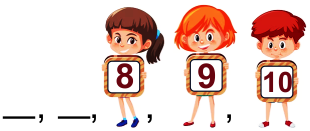Q.2 Find the difference: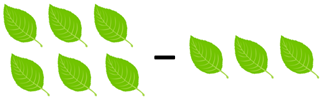Q.3 What is the time in the given clock?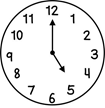Q.4 Find the sum of next two numbers in the pattern: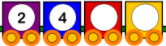Q.5 What is the shape of the given image?Q.6 Find the missing number: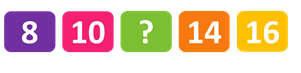Q.7 How many months are there in a year?
 Q.8 Identify the bird which is facing towards right:
 Q.9 Which clock indicates 6:00?
 Q.10 Which one is the back image of the given image: